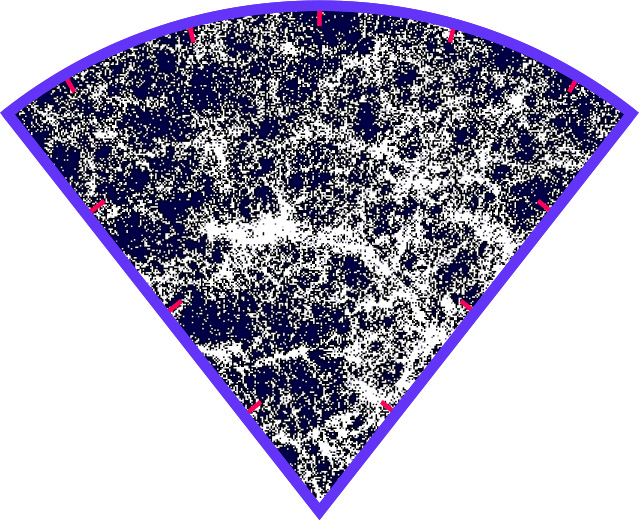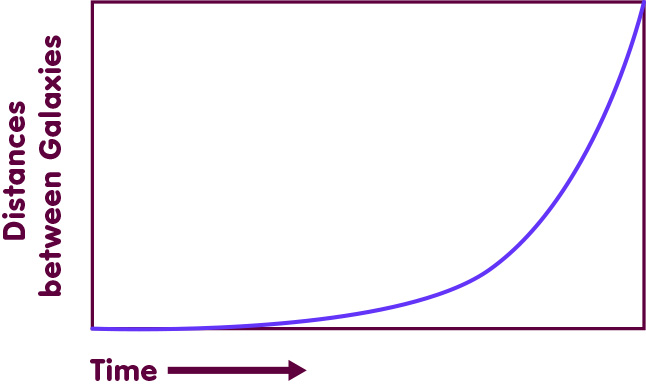# The mathematical universe

### Why cosmology is not only a matter for astronomers and physicists, but also for mathematicians

#### An article by Alan Rendall

Cosmology, the study of the universe as a whole, is not only a matter for astronomers and astrophysicists, but also for mathematicians. At the heart of cosmology, there’s mathematics, and to comprehend the universe, it is not only necessary to observe and measure it, but also to understand its inner workings; it takes not only astronomical data, but also a good understanding of the equations that govern the evolution of the cosmos.

## Initial values and evolution

The appropriate physical theory for describing our universe and its gravitational interactions is general relativity. In addition, one needs to take into account models for the properties of matter such as gas and electromagnetic radiation. Together, this defines physical laws which constrain the properties of the cosmos, equations that any universe must obey. A universe that does satisfy the constraints is, in mathematical parlance, a solution to these equations, just as x=-2 and x=4 are solutions to the quadratic equation x2 – 2·x-8=0. In general relativity, the gravitational field is described by the Einstein equations and the full system may be referred to as the Einstein-matter equations. These equations determine how a given universe evolves in time, or more precisely: they determine the rate of change of important physical quantities, given their values now.

Whenever the values of these quantities are known at some particular time (the “initial values”), the equations make it possible to determine them at all later times. The determination of solutions in this way, the so-called initial value problem, allows solutions to be specified in a controlled way. It is like having a catalogue of all available solutions of the equations. When specific cosmological models are needed for comparison with astronomical observations, they can be looked for in this catalogue. Mathematics provides the building blocks so that astrophysicists can construct their descriptions of the world.

However, to obtain general knowledge about the equations and their solutions is a difficult mathematical problem which, in our present state of knowledge, is not amenable to a complete solution. One way of making progress is to look at model problems that are especially simple, namely those that contain only very simple forms of matter and pose very special initial values.

## Homogeneous universes

A very basic simplification is suggested by astronomical observations: our universe is approximately homogeneous (it is the same at all points of space). As an example, see the following image using data from the 2dF Galaxy Redshift Survey. We, the observers, are at the lower end of the wedge. The wedge-shaped region shows that only part of the sky was searched. Every white dot represents a galaxy, some of them closer to Earth (closer to the sharp end of the wedge), some of them farther away. The greatest distance shown, that of galaxies at the upper boundary, corresponds to a distance of 2 billion light years:To be sure, the distribution of galaxies shows a characteristic porous structure, with filaments of higher and regions of lower density. However, subdividing space into cubes a few hundred light-years across, the average density of each cube is the same – on large scales, the universe is homogeneous.

A suitable model universe, simple yet not too far removed from reality, can be obtained by assuming the universe to be exactly homogeneous. Such models are relatively easy to analyse mathematically. Most predictions of modern cosmology make use of these simple models. Yet it is important to remember that they are simplifications. This brings up a question well-known to mathematicians, that of the stability of particular solutions: Is a certain solution a very special case, or is it representative of the more general situation which is the ultimate goal of study? In other words: If some of the assumptions of a special model are relaxed, say, if regions of slightly higher or lower density are introduced into an initially homogeneous universe, does the modified universe behave in a completely different way? Or is the evolution of the simplified model still a good guide to the evolution of the more realistic, slightly inhomogeneous universe? This leads, in particular, to the investigation of initial values which are close to those for a known situation with high symmetry.

## De Sitter’s cosmos

A specific example of these general considerations is provided by the situation of accelerated cosmological expansion, which may be associated with a positive cosmological constant or, more generally, with dark energy. There is an explicitly known solution of the Einstein equations with positive cosmological constant and without matter. This is the de Sitter solution, first formulated in 1917, a useful simplified model for a universe with accelerating expansion. Adding homogeneous matter suitable for describing the distribution of galaxies does not greatly alter its qualitative properties – at least with respect to this modification, the solution is stable. Thus, although matter is essential in modelling the cosmos, a matter-free solution can be of great relevance.

In a solution of this kind the distance between galaxies increases exponentially with time, as shown in the following graph:It was proved in 1986 that the de Sitter solution is stable in other respects, at least in the absence of matter: If we disturb its homogeneity by adding weak gravitational waves, corresponding to slight inhomogeneous distortions of the homogeneous spacetime, the result is still an exponentially expanding universe which, at late times, comes closer and closer to the undisturbed de Sitter solution.

To find a similar proof of stability for accelerating universes containing matter is currently an open problem. Heuristic arguments indicate that the presence of matter does not fundamentally change the nature of de Sitter expansion, a statement often referred to as the “cosmic no hair theorem”. The interest of proving this stability is evident, as our own universe falls into this class of universes. Stability would not only provide a rigorous justification for the simplified models currently used. It would also mean that, as in the case without matter, universes like ours should come closer and closer to homogeneity as time goes by. This provides a way of understanding how a homogeneous universe like our own can arise over the course of cosmic evolution, without the need for very special initial conditions. However, the proof used for the matter-free case is not easy to adapt to the new situation, and poses something of a challenge. So far, a proof has only be found for certin cases with symmetry.

At the same time models are being studied where, in addition to ordinary matter representing the galaxies, there is exotic matter with negative pressure known as quintessence – another way of obtaining accelerated expansion, distinct from Einstein’s cosmological constant. Since it is not known in detail what is behind the tendency of our universe to accelerate, it makes sense to keep all options open and study the mathematical properties of large classes of models for dark energy.

## Further Information

For the relativistic ideas behind this spotlight topic, check out Elementary Einstein, especially the chapters General Relativity and Cosmology.

Related Spotlights on relativity can be found in the sections General relativity and Cosmology.